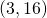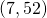# General Term Given Points on a Graph

When graphing a sequence, the horizontal axis is used to represent the place in the sequence (), the vertical axis is used to represent the value of the terms ().

For example, if the pointsandwere plotted on the graph of an arithmetic sequence, we could conclude:We could then proceed as instructed here.